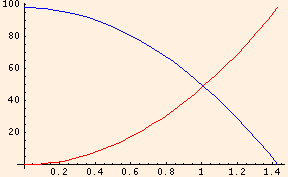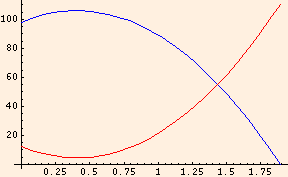# Conservation Principles

Within the confines of our point particle approximation, we have done rather well: given the height from which you jumped, your initial velocity, the time it took you to stop, your mass and the size of the impact area, we have been able to completely describe your fall and its consequences. If this has been your first exposure to physics, it has probably seemed to be a complicated analysis. Yet many if not most of the problems to which physics addresses itself are beyond any such complete treatment.

### Energy

K = m v2 / 2.
Since mass is always positive, we can see that the kinetic energy is also always positive. The S.I. units of energy are clearly kg m2 / s2, which are called Joules (denoted J). As is obvious, a one kg mass moving at one meter per second has one half Joule of kinetic energy.

Potential energy is the energy an object has by virtue of its position in the presence of an external force. When you were on the roof attempting to clean the gutters you had a higher potential energy than when you were on the ground. Potential energy is always relative, so that it is necessary to fix a point at which it is zero before we can decide whether it is positive or negative at any given position. In the case of your gravitational potential energy in the presence of the Earth's gravitational attraction, we typically say that the potential energy is zero at ground level.

U = m g y
= m g (h - g t2 / 2)

= m g h - m g2 t2 / 2

K = m v2 / 2
= m (-g t)2 / 2

= m g2 t2 / 2

And since we are ignoring air resistance, the sum of the two was a constant (m g h) at every point along the path of your fall:Here we have plotted the kinetic energy in red and the potential energy in blue.

When you jumped, you had an initial kinetic energy equal to

K0 = m v02 / 2
= m (vx02 + vy02) / 2
(from the Pythagorean Theorem), and your kinetic and potential energies were
K = m v2 / 2
= m (vx02 + (vy0 - g t)2) / 2

= m v02 / 2 + m g2 t2 / 2 - m g vy0 t

and
U = m g y
= m g (h + vy0 t - g t2 / 2)

= m g h + m g vy0 t - m g2 t2 / 2.

Their sum is m v02 / 2 + m g h throughout your trajectory:Note that the kinetic energy has a minimum precisely where the potential energy is a maximum, but that it is never zero. This corresponds to the vertical component of your velocity dropping to zero at the height of your jump, while the horizontal component of your velocity is constant throughout.

In your actual collision with the Earth, energy was not conserved. It was dissipated, both in the compression of the ground and the creation of your injuries. Energy is only conserved in collisions when they are perfectly elastic. This only happens in cartoons, although it is a decent approximation when the colliding objects are not deformed. When energy is expended (such as in your collision), we can define the rate at which it is expended as the power. Power is denoted by the letter P, as is pressure; you must distinguish between the two based on the context (this will happen again: there are not enough letters in the alphabet to avoid it!). The S.I. unit of power is the kg m2 / s3, otherwise known as the Watt (denoted W). This is the same Watt that you are familiar with in light bulbs, where the energy being expended is electrical.

Note that in all of this discussion, energy is an attribute, much like mass, or even velocity or position. Energy is not a substance, although many texts treat it as such. It is a concept of profound use in physics: physicists are always asking questions like "what is the configuration of least energy?", or "where was the energy transported to?", in order to learn more about the behavior of a system.

### Momentum

Just before you hit the ground, your fall had given you a rather large momentum. After you came to rest, your momentum was now zero. But that momentum was not lost: it was transferred to the Earth, which now has a slight velocity along the direction of your fall. As in the case of your mutual gravitational attraction with the Earth, this velocity is negligible, because the mass of the Earth is much greater than yours.

Notice that an external force can change the momentum of an object. This should of course be obvious, since a force implies acceleration and acceleration implies a change in velocity.

You now have some work to do. The following applet is like the one you used to construct the equations of kinematics. You will use it to construct equations involving energy, momentum and power, which you will then use in the second applet below.

You need a Java-capable browser to be able to use the applets. If they do not work with your Windows system, download the Java VM (Virtual Machine) for your version of Windows at the download section at java.sun.com.

You need a Java-capable browser to be able to use the applets. If they do not work with your Windows system, download the Java VM (Virtual Machine) for your version of Windows at the download section at java.sun.com.

In the next section we will apply what we have learned here to see why friction is so important to your everyday life.